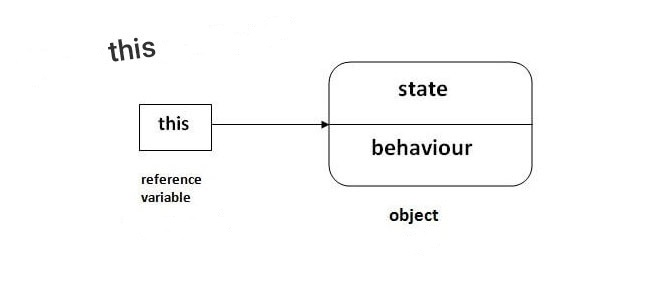# blog posts# In this tutorial, you will learn the keyword this in Java; You will learn how and where to use it with the help of an example.

## This keyword

In Java, this refers to the current object in methods or constructs. Let’s give an example to prove it.

1. class MyClass {
2. int instVar;
3. MyClass (int instVar) {
4. this.instVar = instVar;
5. System.out.println (“this reference =” + this);
6. }
7. public static void main (String [] args) {
8. MyClass obj = new MyClass (8);
9. System.out.println (“object reference =” + obj);
10. }
11. }

Output

this reference = com.ThisAndThat.MyClass@74a14482

object reference = com.ThisAndThat.MyClass@74a14482

Note that the obj and this object identifiers are the same. This means nothing more than referring to the current object.

There are 3 situations in which this keyword is commonly used.

### 1. Use this to separate different variable sources

In Java you are not allowed to define two or more variables with the same name in a domain (class domain or method domain). However, the sample variables and parameters may have the same name. As:

class MyClass {

int instVar; // instVar instance variable

MyClass (int instVar) {// instVar parameter

instVar = instVar;

}

}

In the above program, the Java compiler is mistaken due to the ambiguity of the name. Therefore, to solve this problem, the keyword this is used.

Let’s look at an example without using this keyword:

1. class MyClass {
2. int instVar;
3. MyClass (int instVar) {
4. instVar = instVar;
5. }
6. public static void main (String [] args) {
7. MyClass mc = new MyClass (8);
8. System.out.println (“mc.instVar =” + mc.instVar);
9. }
10. }

#### Output

mc.instVar = 0

You might expect the output to be 8, but instead printed 0 because the Java compiler makes a mistake due to the name ambiguity between the sample variable and the constructor parameter.

Now, let’s rewrite the above code and use this keyword to solve this problem.

1. class MyClass {
2. int instVar;
3. MyClass (int instVar) {
4. this.instVar = instVar;
5. }
6. public static void main (String [] args) {
7. MyClass obj = new MyClass (8);
8. System.out.println (“obj.instVar =” + obj.instVar);
9. }
10. }

#### Output

mc.instVar = 8

You will now get the expected output. Because when you create an object, the Java compiler knows which object the constructor used.

When the Java compiler calls the constructor, this is replaced inside the constructor by the object that called the constructor.

Note: If you submit a parameter that has a different name than the sample variables, the compiler will automatically attach the this keyword.

This code

class MyClass {

int instVar;

MyClass (int i) {

instVar = i;

}

}

#### Is equal to:

class MyClass {

int instVar;

MyClass (int i) {

this.instVar = i;

}

}

#### Another common use of this keyword is in the get and set methods of a class. For example:

1. class POJO {
2. String name;
3. void setName (String name) {
4. this.name = name;
5. }
6. String getName () {
7. return this.name;
8. }
9. public static void main (String [] args) {
10. POJO pojo = new POJO ();
11. pojo.setName (“Toshiba”);
12. System.out.println (“pojo.name:” + pojo.getName ());
13. }
14. }

Output

pojo.name: Toshiba

When working with builders overloading, it may be more useful to call one builder than another. However, the creators can not be explicitly called. Therefore, you can use another form of this keyword to achieve this goal. (() this)

The pseudo-code is shown here:

this (arg-list)

Here’s how to use this in the manufacturer’s call.

1. class Complex {
2. private int a, b;
3. // parameterize constructor
4. private Complex (int i, int j) {
5. this.a = i;
6. this.b = j;
7. }
8. private Complex (int i) {
9. this (i, i); // invokes Complex (int i, int j);
10. }
11. private Complex () {
12. this (0); // invokes Complex (int i);
13. }
14. @Override
15. public String toString () {
16. return this.a + ”+” + this.b + “i”;
17. }
18. public static void main (String [] args) {
19. Complex c1 = new Complex (2, 3);
20. Complex c2 = new Complex (3);
21. Complex c3 = new Complex ();
22. System.out.println (c1);
23. System.out.println (c2);
24. System.out.println (c3);
25. }
26. }

#### Output

2 + 3i

3 + 3i

0 + 0i

In the above program, it does not matter which constructor is called when introducing the object, finally the parametric constructor is called.

Be careful when using this (). Constructors calling this () run slowly because calling an additional constructor adds another overhead. If your class is only used to create a handful of objects, using this () is useful. Another great advantage of using this () is the reduction of duplicate code.

### 3- Send this as an argument

If you need to move the current object as an argument to a method, you can use this.

1. class ThisExample {
2. int x;
3. int y;
4. ThisExample (int x, int y) {
5. this.x = x;
6. this.y = y;
7. System.out.println (“Before passing this to addTwo () method:”);
8. System.out.println (“x =” + this.x + “, y =” + this.y);
10. System.out.println (“After passing this to addTwo () method:”);
11. System.out.println (“x =” + this.x + “, y =” + this.y);
12. }
13. void addTwo (ThisExample o) {
14. ox + = 2;
15. oy + = 2;
16. }
17. }
18. class Demo {
19. public static void main (String [] args) {
20. ThisExample obj = new ThisExample (1, -2);
21. }
22. }

#### Output

Before passing this to addTwo () method:

x = 1, y = -2

After passing this to addTwo () method:

x = 3, y = 0

Here, the addTwo () method is called with this as an argument from within the constructor.